ICSE Class 9 Complementary Angles Solution New Pattern

## ICSE Class 9 Complementary Angles Solution New Pattern By Clarify Knowledge

ICSE Class 9 Complementary Angles Solution New Pattern 2022

## Chapter 25 - Complementary Angles Exercise Ex. 25

Question 1(i)

Solution 1(i)

Question 1(ii)

Solution 1(ii)

Question 1(iii)

Solution 1(iii)

Question 1(iv)

Solution 1(iv)

Question 1(v)

Solution 1(v)

Question 1(vi)

Solution 1(vi)

Question 1(vii)

Solution 1(vii)

Question 1(viii)

Solution 1(viii)

Question 2(i)

Solution 2(i)

Question 2(ii)

Solution 2(ii)

Question 2(iii)

Solution 2(iii)

Question 2(iv)

Solution 2(iv)

Question 2(v)

Solution 2(v)

Question 2(vi)

Solution 2(vi)

Question 3(i)

Show that:

tan 10° tan 15° tan 75° tan 80° = 1Solution 3(i)

L.H.S.

= tan 10° tan 15° tan 75° tan 80°

= tan (90° - 80°) tan (90° - 75°) tan 75° tan 80°

= cot 80° cot 75 ° tan 75° tan 80°

= (cot 80° tan 80°)(cot 75° tan 75°)

= (1)(1)

= 1

= R.H.S.Question 3(ii)

Show that:

sin 42° sec 48° + cos 42° cosec 48° = 2Solution 3(ii)

Question 4

Express each of the following in terms of angles between 0°and 45°:

(i) sin 59°+ tan 63°

(ii) cosec 68°+ cot 72°

(iii)cos 74°+ sec 67°Solution 4

Question 5

For triangle ABC, show that:

(i)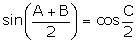(ii)Solution 5

(i) We know that for a triangleABC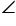A +B +C = 180°

(ii) We know that for a triangleABCA +B +C = 180°B +C = 180° -A

Question 6

Evaluate:

(i)(iii)(iv)(v)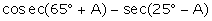(vi)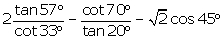(vii)(viii)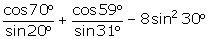(ix)Solution 6

(i)

(iii)(iv)(v)(vi)(vii)(viii)(ix)Question 7

Solution 7

Question 8(i)

In each case, given below, find the value of angle A, where 0° ≤ A ≤ 90°.

sin (90° - 3A).cosec 42° = 1Solution 8(i)

Question 8(ii)

In each case, given below, find the value of angle A, where 0° ≤ A ≤ 90°.

cos (90° - A).sec 77° = 1Solution 8(ii)

error: Content is protected !!